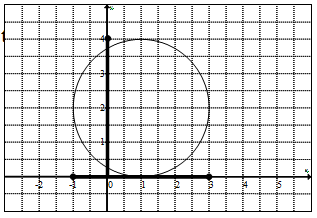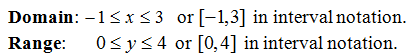## Pages

### Relations, Graphs, and Functions

In this section we will study functions in more depth and begin by defining a relation as any set of ordered pairs.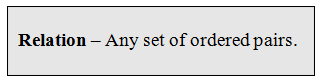An example of a relation might look like { (2,3), (2,5), (0,1) }. Here we have a relation consisting of three ordered pairs or points on the Cartesian coordinate system. We are familiar with ordered pairs and usually see them denoted as (x, y).  Typically the x-value (the first component) will be the independent variable or input and the y-value (the second component) is the dependent variable or output.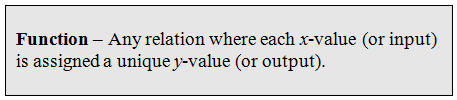The example { (2,3), (2,5), (0,1) } is NOT a function because the x-value 2 is assigned more than one y-value, namely 3 and 5. For every x-value there can be only one y-value. Next we define the domain as the set of x-values and the range as the set of y-values for which the relation is defined.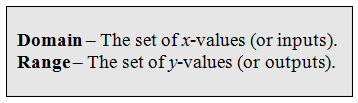Example:Tip: When looking at a list of ordered pairs, if there are repeating x-values then the relation is not a function.  This usually indicates that there is an input with multiple outputs. (This does not apply to the y-values)

Determine if the relations are functions.  If so state the domain and range.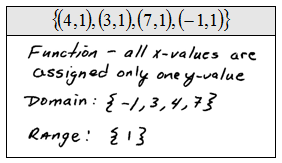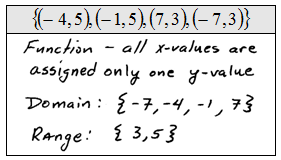Relations can consist of an infinite number of ordered pairs, in which case, making a big list would be impossible. A graph can represent a relation by considering it as a big list of ordered pairs.  Each dot on the graph represents an ordered pair (x, y).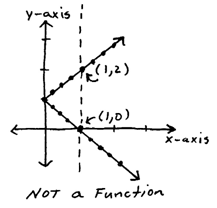Notice that if we can draw a vertical line that intersects the graph twice we will be able to identify one x-value with two corresponding y-values.  Therefore, it can not be a function.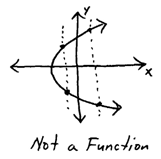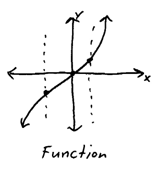Alternatively, if any vertical line crosses the graph only once then it does represent a function.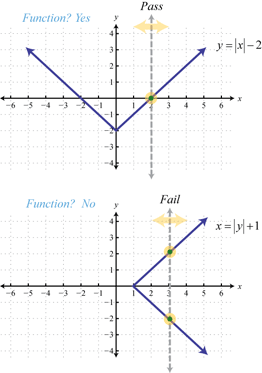Use the vertical line test to determine whether or not the graph represents a function.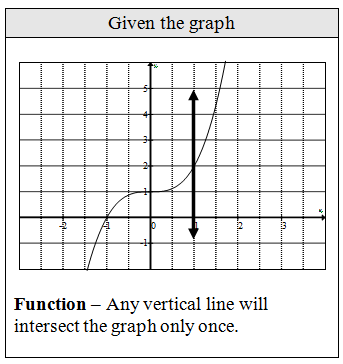Be prepared to state the domain and range given the graph.  Remember to think of the graph as an infinite set of ordered pairs (x, y).  From the graph, determine the x-values and y-values. First shade in the domain and range then convert to interval notation.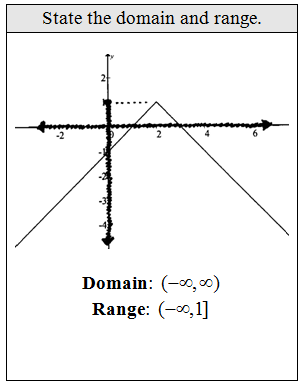It should be clear, at this point, that the circle shown is not a function.  Nonetheless, we still can determine the domain and range of the relation that it represents.